# 2021 P1 Q6 - Deduce Shape and Polarity of Molecules

Here's 2021 A Levels H2 Chemistry Paper 1 Question 6.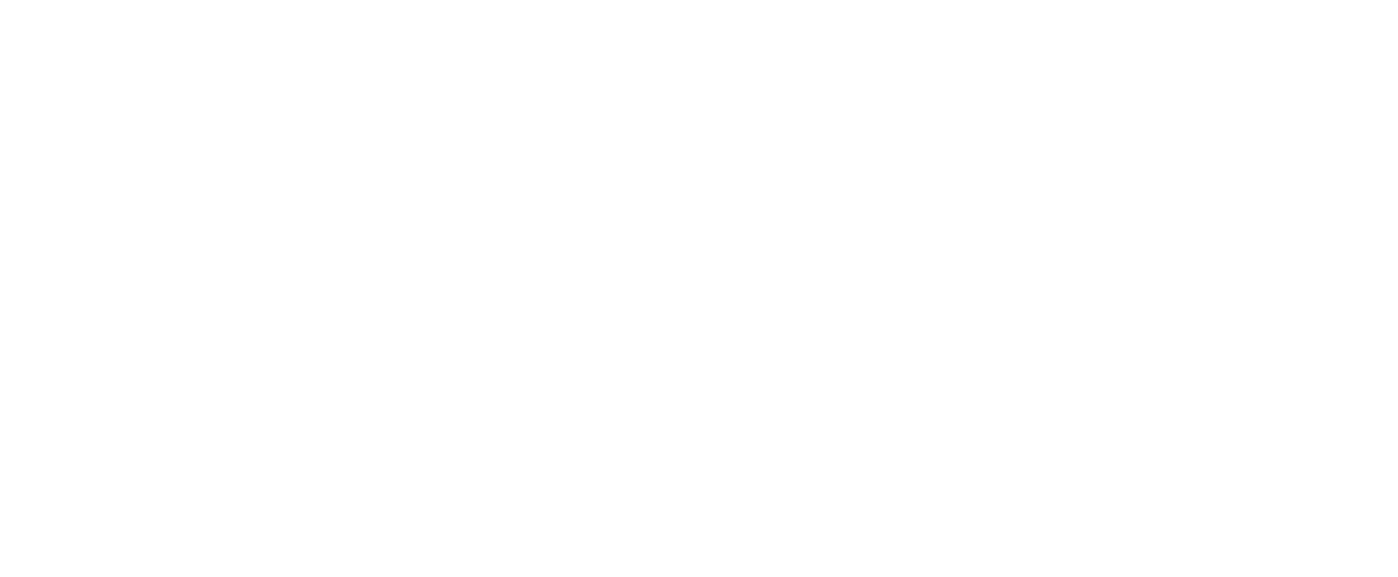We are required to determine which molecule has the correct molecular shape and polarity.

For each option, we need to:

- consider the dot and cross diagram to determine the number of bond pairs and lone pairs with respect to the central atom
- deduce the shape using VSEPR theory
- determine if the molecule is polar or non-polar

Let's take a look at each of these molecules.

A. Boron trichloride, BCl3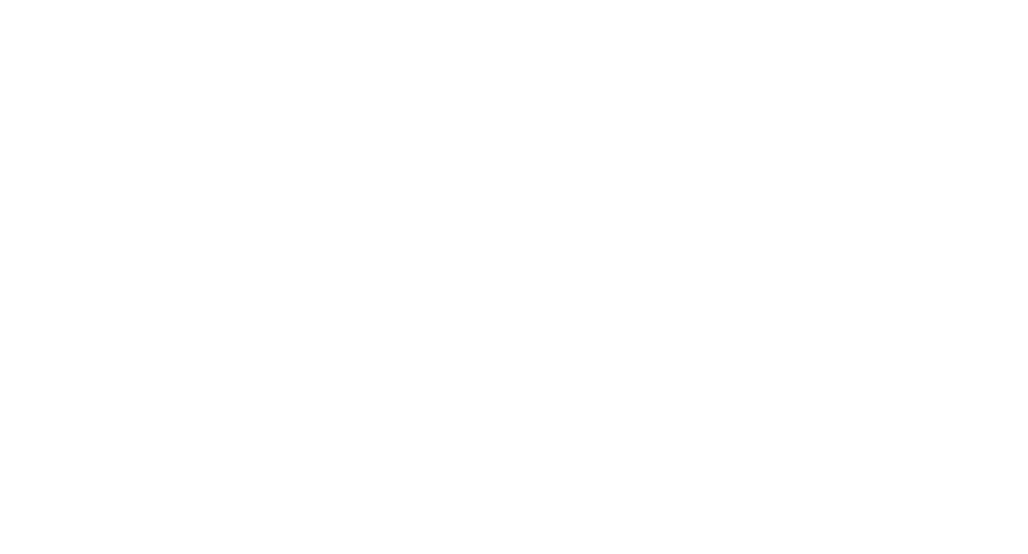Central atom B has 3 bond pairs and no lone pair.

Shape is trigonal planar which is correct.

B-Cl bond is polar but the dipole moments from these 3 B-Cl bonds will cancel out exactly due to the high symmetry of trigonal planar shape.

Hence BCl3 is non-polar and option A is not correct.

B. Nitrogen trichloride, NCl3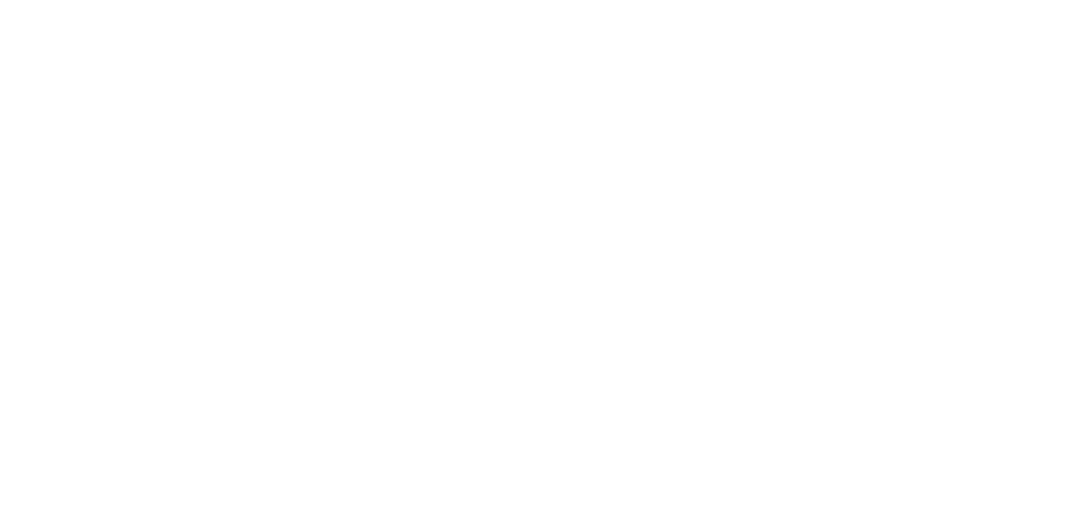Central atom N has 3 bond pairs and 1 lone pair.

Shape is trigonal pyramidal which is correct.

N-Cl bond is polar and the dipole moments from these 3 N-Cl bonds will have a net dipole moment pointing upwards.

Hence NCl3 is polar and option B is not correct.

C. Sulfur dioxide, SO2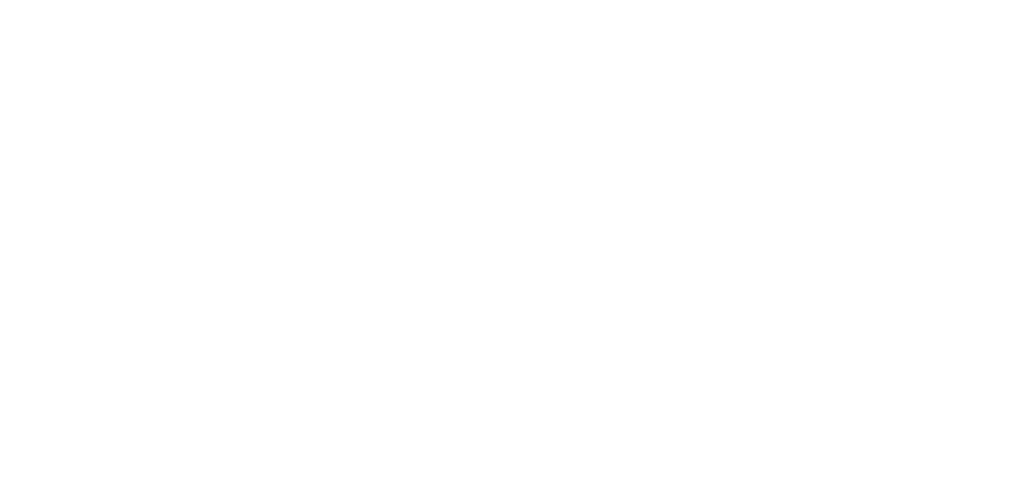Central atom S has 2 bond pairs and 1 lone pair.

Shape is bent and hence option C is not correct.

D. Trichloromethane, CHCl3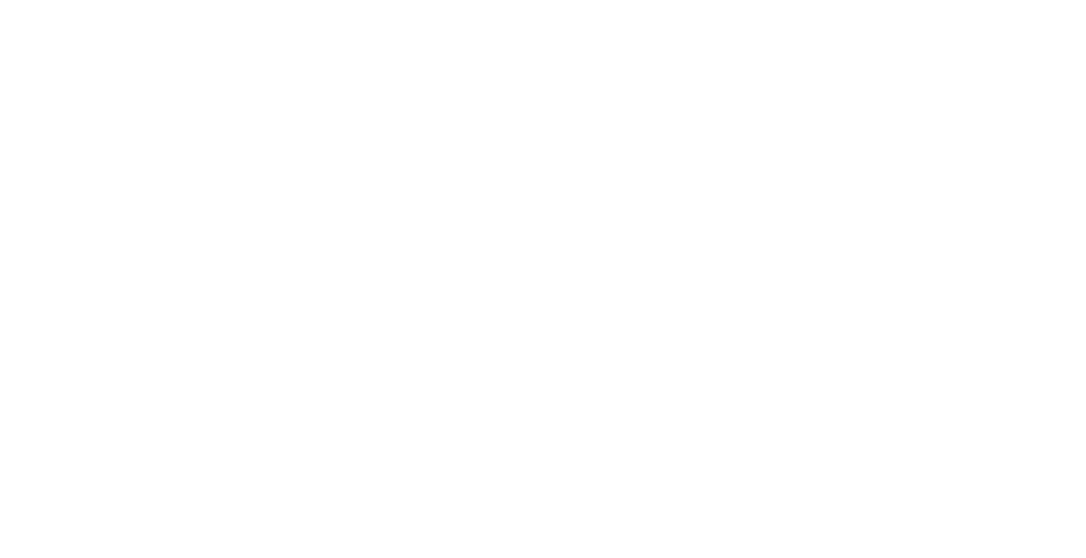Central atom C has 4 bond pairs and no lone pair.

Shape is tetrahedral which is correct.

C-Cl bond is polar and C-H bond is not polar. The dipole moments from the 3 C-Cl bonds will have a net dipole moment pointing downwards.

Hence CHCl3 is polar and option D is correct.

Therefore the answer to this question is option D.

Topic: Chemical Bonding, Physical Chemistry, A Level Chemistry, Singapore

Back to list of questions for 2021 A Level H2 Chemistry Paper 1.

Found this A Level Chemistry video useful?